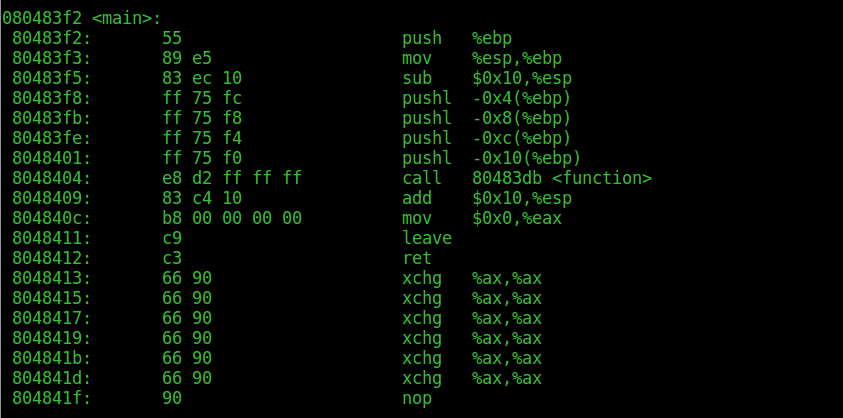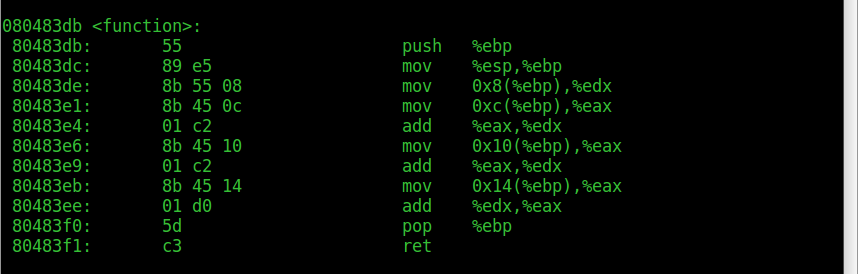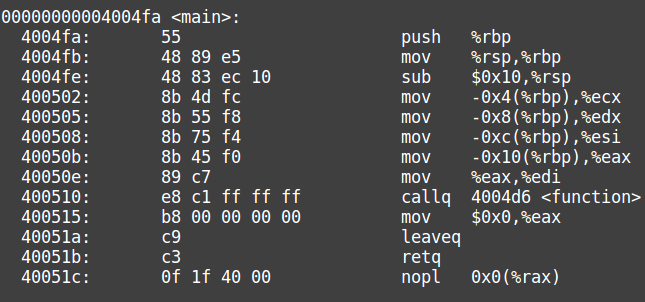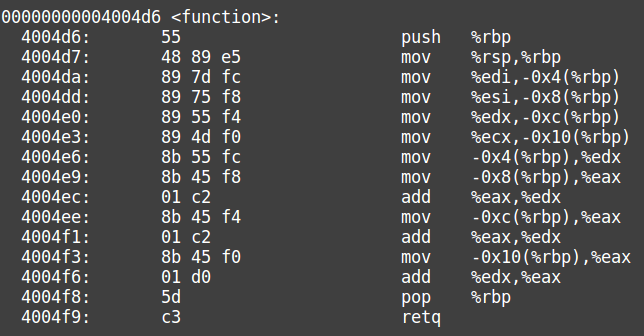In the first post on my new website I want to talk about something about which is not really easy to find information: the calling convention in 64-bit machines. First things first, what is a calling convention and why it is important in the security world?

The calling convention, in short, describes how functions are called, how parameters are passed to such functions and how results are collected. This matters a lot when dealing with buffer overflows, for example, let’s see why.

### Why it matters

While reading about buffer/stack overflows, it is common to refer to 32-bit architectures, this happens for example in The Shellcoder’s Handbook or in Grey Hat Hacking Fourth Edition and in all the binary exploitation books I have found so far. This means that, when the stack usage is introduced, the information refers to such systems and in particular the layout of the stack frame is quite different compared to what it is possible to test natively on current systems. Let’s explore the amd64/x64 calling convention by showing the differences with the x86 one.

### x86 v x64 calling convention

A major difference between 32 and 64-bit machines is the number of available registers. In modern architectures in fact, these come not only in bigger size, but also in bigger amount. This is in fact the reason behind the biggest difference between the two calling convention. Let’s see what happened in a 32-bit machine:

Let’s assume the code is the following:

``````    int function(int a, int b, int c, int d){
return a+b+c+d;
}

int main() {
int a, b, c, d;
function(a, b, c, d);
}
``````

If we compile this piece of code with

``````gcc -m32 -o code1 code1.c
``````

we can see that main function has been compiled as follow:What happened? Well, the textbook procedure. Before the function ‘function’ is called, its parameters, in this case a,b,c and d, are prepared on the stack. In this case they are all 0s, so it’s not really fun, but it helps not ‘polluting’ the code with other instructions. As we can clearly see, there are 4 pushl instructions, respectively to -0x4 up to -0x10 (16 bytes) respect the base pointer of the main function. We have to remember that the stack grows ‘backwards’, that means that the top of the stack is at lower addresses. These instructions clearly push the 4 integers passed to function on the stack, in right-to-left order, so that a will be on top and can be popped as first.

This is nothing else than the standard way to call a function in 32-bit architectures. First, the space is allocated on the stack, with the sub \$0x10, %esp, which creates 16 bytes of space on the stack by moving the stack pointer. Then, the parameters are pushed one by one. After this, the call instruction will set the next instruction address on the stack as well and will pass the control to function.Function then retrieves the parameters starting from 0x8(%ebp) up to 0x14(%ebp). This is because at 0x4(%esp) there is the return address, aka 0x8048409, the address of the next instruction in main after call. As we can see, the result is places in %eax.

### The 64bit case

Now let’s observe what happens if we compile the code for 64-bit architectures.

Let’s compile the same exact code, omitting the -m32 flag.

``````gcc -o code64bit code1.c
``````

Now let’s use objdump to inspect the main function.The code is not really clear, but we can see that after the prologue the stack pointer is moved 16 bytes in memory. After this, the values of the bytes just added to the stack are added respectively in %ecx, %edx,%esi,%eax and this last one is then put in %edx.

After this, function is called. Let’s see what function does.We can cleary see from the code that this time the parameters are taken from %edi,%esi,%edx,%ecx and then they are summed together.

This is probably the biggest change in the calling convention in 64-bit architectures.

### Calling convention in x64

In order to learn about this, personally I found only one good resource, the Application Binary Interface, that you can download for example here . This is not really a friendly read, but has all the necessary information. A shorter document on x64 in general where there is some information also about the calling convention is this cheat sheet. Let’s try to summarize finally what is the calling convention for amd64 systems.

After the parameters for a function are computed, they are classified. There are quite a few categories, including SSE, X87 and few others, but the most interesting are INTEGER and MEMORY. A parameter is of class INTEGER if it is a type _Bool, char, short, int, long and long long or they are pointers. A parameter is of class MEMORY if its size is bigger than 8bytes or if it is not aligned in memory (note that there are bigger arguments, such as _m512 that have their own category and therefore even being bigger than 8 bytes are not classified as MEMORY) After the classification, arguments of type MEMORY are placed on the stack exactly as it happened in 32-bit architectures, and this happens in C order, meaning from right to left. The first parameter of a function has the lowest address. Arguments of type INTEGER are placed in the first free register among %rsi, %rdi, %rcx, %rdx, %r8, %r9. If there are more than 6 arguments, the stack is used.

The result of a function is collected as follows:

• If the type of the return is MEMORY, then the caller uses %rdi to pass to the callee the address in which expects such result (hidden argument). The callee then doesn’t do anything else than returning this same address in %rax.
• If the type of the return is INTEGER, then the return value is stored in the first available register among %rax and %rdx.

### Conclusion

I want to note that the convention does not change that much if you are studying security, but knowing it helps testing buffer overflows on actual machines, without compiling code for 32 bit systems, that nowadays are a small minority. One common case in fact is a description of the stack frame that in many cases (especially the simplest used in some books) is completely different from what it is possible to get on your computer. This happens, or at least happened to me, since I learned the textbook version of the arguments passed on the stack without diving into real life cases, on 64bit architectures. Since understanding the stack frame is the first step to understand how the stack overflow works, I think it’s important to have some better understanding of what happens in the current machines.

Clearly there is much more than what is written here, and if you are interested in cases involving programs with SSE arguments or other advanced topics, I invite you to read the ABI linked above. What I tried is to give a relatively simple overview of what a calling convention is and how it works for 64-bit architectures. The main aim of this article was getting started someone who wants to study binary attacks or reverse engineering, without the need to dig into the unfriendly ABI.

For every correction, comment, suggestion or whatever you might have in mind, feel free to drop a mail to: security[at]coolbyte[dot]eu.